CHAPTER 10:

AN INTRODUCTION TO KINETICS AND EQUILIBRIUM

The following information is often presented on the first page of exams covering this chapter under the heading: “potentially useful information.”

[X] = concentration of X at equilibrium, in moles per liter

(X) = concentration of X at any moment in time, in moles per liter

CX= initial concentration of X, in moles per liter

Writing Equilibrium Constant Expressions

10-1. What is the correct equilibrium constant expression for the reaction:

Cl2(g) + 3 F2(g) ⇌ 2 ClF3(g)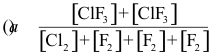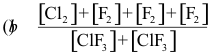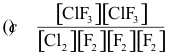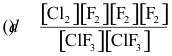Answer: (c)

10-2. What is the correct equilibrium constant expression for the reaction:

2 NOCl(g) ⇌ 2 NO(g) + Cl2(g)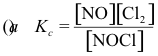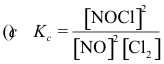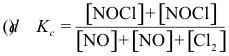Answer: (e)

10-3. What is the correct equilibrium constant expression for the reaction:

2 NO2(g) ⇌ 2 NO(g) + O2(g)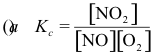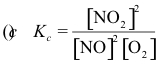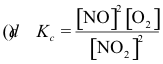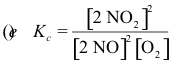Answer: (d)

Combining Equilibrium Constant Expressions

10-4. Assume that the equilibrium constants for the following reactions are known.

2 SO2(g) + O2(g) ⇌ 2 SO3(g)                K1

2 CO(g) + O2(g) ⇌ 2 CO2(g)                 K2

What is the equilibrium constant for the following reaction?

2 SO2(g) + 2 CO2(g) ⇌ 2 SO3(g) + 2 CO(g)

(a) K = K1 x K2 (b) K = K1/K2 (c) K = K2/K1

(d) K = K1 + K2 (e) K = K1 K2

Answer: (b)

10-5. Assume that the equilibrium constant for the following reaction is known.

2 NO2(g) ⇌ 2 NO(g) + O2(g)                  K1 = 7.4 x 10-16      (at 25°C)

What is the correct value of the equilibrium constant for the opposite reaction?

2 NO(g) + O2(g) ⇌ 2 NO2(g)                   K2 = ?

(a) K2 = K1 = 7.4 x 10-16 (b) K2 = 1/K1 = 1.4 x 1015

(c) K2 = K1(RT) = 1.8 x 10-14 (d) K2 = K1(RT)-1 = 3.0 x 10-17

(e) impossible to determine from this information

Answer: (b)

10-6. Assume that Kp = 1.75 atm for the reaction.

CO2(g) + C(s) ⇌ 2 CO(g)

Calculate Kp for the following reaction.

CO(g) ⇌ ½ CO2(g) + ½ C(s)

(a) 0.756 (b) 1.32 atm (c) 1.75 atm (d) 3.06 atm

(e) none of the above

Answer: (a)

Calculating Equilibrium Constants

10-7. The composition of the following system at equilibrium at 1000 K is 0.202 atm CO2, 0.594 atm CO, and 5 kg C.

2 CO(g) ⇌ CO2(g) + C(s)

What is the equilibrium constant for this reaction?

(a) 2.94 (b) 14.56 (c) 0.572 (d) 0.337 (e) none of the above

Answer: (c)

10-8. For the reaction: CO(g) + Cl2(g) ⇌ COCl2(g)

kf is 1 x 107 M-1 s-1 and kr = 2 x 102 s-1. What is the equilibrium constant for the reaction?

(a) 2 x 10-5 (b) 4.70 (c) 5 x 104 (d) 2 x 109 (e) none of the above

Answer: (c)

10-9. The following reaction is used to produce a mixture of CO and H2 known as synthesis gas.

C(s) + H2O(g) ⇌ CO(g) + H2(g)

Assume that 1 atm of H2O(g) and excess solid carbon are sealed in a 1.0 L container at 700°C. After the reaction reaches equilibrium, the total pressure in the container is 1.65 atm. What is the equilibrium constant for this reaction?

Answer: 1.21

General Equilibrium Questions

10-10. Which statement concerning the following reaction isn’ true?

2 HI(g) ⇌ H2(g) + I2(g)

(a) At equilibrium, the concentrations of the three gases do not change with time.

(b) Kp = Kc

(c) The partial pressures of I2 and H2 re equal at equilibrium, regardless of the initial pressures of HI, H2, and I2.

(d) HI decomposes as fast as it is formed once equilibrium is established.

(e) The percent dissociation of HI does not depend on the total pressure.

Answer: (c)

10-11. The reaction:

O(g) + H2O(g) ⇌ CO2(g) + H2(g)

has an equilibrium constant K = 5.0 at a certain temperature. A 1.00-L vessel contains 0.20 mole of CO, 0.30 mole of H2O, and 0.90 mole of H2 in equilibrium at this temperature. What is the expression for K in terms of the concentrations of the components? What is the concentration of CO2 in this equilibrium mixture?

Answer: [CO2] = 0.333 M

Relationship between Kc and Kp

10-12. What is the relationship between Kp and Kc for the following reaction?

Cl2(g) + 3 F2(g) ⇌ 2 ClF3(g)

(a) Kc = Kp (b) Kc = Kp x (RT)-1 (c) Kc = Kp x (RT)-2

(d) Kc = Kp x (RT) (e) Kc = Kp x (RT)2

Answer: (e)

10-13. The correct value for the equilibrium constant Kp for the reaction

2 NO2(g) ⇌ 2 NO(g) + O2(g)       Kc = 7.4 x 10-16

would be:

(a) K = Kc = 7.4 x 10-16 (b) Kp = 1/Kc = 1.4 x 1015

(c) Kp = Kc (RT) = 1.8 x 10-14 (d) Kp = Kc (RT)-1 = 3.0 x 10-17

(e) none of the above.

Answer: (c)

14. If Kc = 5.6 x 10-6 for the following reaction at 400 K

NOCl(g) ⇌ 2 NO(g) + Cl2(g)

what is the value of Kp for this reaction?

(a) 1.7 x 10-7 (b) 5.6 x 10-6 (c) 1.8 x 10-4

(d) 6.0 x 10-3 (e) 1.8 x 105

Answer: (d)

15. The correct value for the equilibrium constant for the reaction

2 NO2(g) ⇌ 2 NO(g) + O2(g)                   Kc = 7.4 x 10-16       (at 25°C)

expressed in terms of the partial pressures of NO2, NO and O2 for this reaction would be:    (a) Kp = Kc (b) Kp = 1/Kc (c) Kp = Kc(RT)

(d) Kp = Kc(RT)-1 (e) none of the above

Answer: (c)

16. What is the value of “n” in the following equation

Kp = Kc(RT)n

for the reaction

2 C2H6(g) + 7 O2(g) ⇌ 4 CO2(g) + 6 H2O(g)

(a) -l (b) 0 (c) 1 (d) 2 (e) 3

Answer: (c)

Relationship Between Qc and Kc

17. If at any moment in time, we find that Qc is larger than Kc for the reaction:

Cl2(g) + 3 F2(g) ⇌ 2 ClF3(g)

we can conclude that:

(a) The reaction is at equilibrium.

(b) The reaction cannot reach equilibrium.

(c) The reaction must shift to the right to reach equilibrium.

(d) The reaction must shift to the left to reach equilibrium.

(e) None of the above are possible.

Answer: (d)

10-18. Assume that the reaction quotient, Qc, for the following reaction at 25°C is 1.0 x 10-8

2 NO2(g) ⇌ 2 NO(g) + O2(g)                 Kc = 7.4 x 10-16       (at 25°C)

From this we can conclude:

(a) The reaction is at equilibrium.

(b) Equilibrium could be reached by adding enough NO or O2 to the system.

(c) The reaction must proceed from left to right to reach equilibrium.

(d) The reaction must proceed from right to left to reach equilibrium.

(e) The reaction can never reach equilibrium.

Answer: (d)

10-19. If the equilibrium constant for the following reaction is Kc = 1 x 102

2 C2H6(g) + 7 O2(g) ⇌ 4 CO2(g) + 6 H2O(g)

and all the concentrations were initially 0.10 M, we can predict that the reaction:

(a) is at equilibrium initially.

(b) must shift from left to right to reach equilibrium.

(c) must shift from right to left to reach equilibrium.

(d) cannot reach equilibrium.

(e) cannot be determined unless we have the information necessary to calculate Qc for the reaction.

Answer: (b)

10-20. What is the correct expression for the reaction quotient for the reaction:

2 NO2(g) ⇌ 2 NO(g) + O2(g)       Kc = 7.4 x 10-16 at 25°C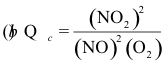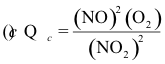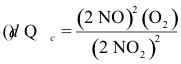(e) none of the above

Answer: (c)

10-21. For the following reaction:

2 NOCl(g) ⇌ 2 NO(g) + Cl2(g) Kc = 5.6 x 10-6 (at 400 K)

If: (NOCl) = 0.0222 M, (Cl2) = 0.0222 M, and (NO) = 0.989 M at some moment in time, we can conclude that:

(a) the reaction is at equilibrium.

(b) some of the NOCl must be converted to NO and Cl2 to reach equilibrium.

(c) some of the NO and Cl2 must be converted to NOCl to reach equilibrium.

(d) it is impossible to determine which way the reaction must go to reach equilibrium.

(e) the reaction can never reach equilibrium.

Answer: (c)

10-22. Suppose that the reaction quotient, Qc, for a chemical reaction was found to be close to unity (Qc ≈ 1). Given this information we can accurately predict:

(a) that the reaction will proceed in a direction to generate more product.

(b) that the reaction will proceed in a direction to use up product.

(c) that the reaction is close to equilibrium.

(d) nothing about the direction the reaction will proceed without additional information.

(e) none of the above.

Answer: (d)

10-23. The equilibrium constant for the following reaction at a given temperature is 8.24 x 104.

2 SO2(g) + O2(g) ⇌ 2 SO3(g)

If the partial pressure of SO2 is 1.2 x 10-5 atm, the partial pressure of O2 is 1 atm, and the partial pressure of SO3 is 1 atm, we can accurately state:

(a) that the reaction is at equilibrium.

(b) that the reaction must shift from left to right to reach equilibrium.

(c) that the reaction must shift from right to left to reach equilibrium.

(d) nothing about the reaction without additional information.

Answer: (c)

10-24. Given the equilibrium constant for the following reaction.

H2(g) + Cl2(g) ⇌ 2 HCl(g)             Kp = 2.5 x 104

If equimolar amounts of H2 and Cl2 are allowed to reach equilibrium in a system in which no HCl was present initially, the ratio of the partial pressures of H2 to Cl2 at equilibrium will be:

(a) larger than 1. (b) smaller than 1. (c) equal to 1.

(d) have a value that can't be generalized by any of the above statements.

Answer: (c)

10-25. For the following reaction:

2 NO2(g) ⇌ 2 NO(g) + O2(g) Kc = 7.4 x 10-16           (at 25°C)

If (NO2) = 0.10 M, (NO) = 0.010 M, and (O2) = 1.0 x 10-5 M, we can correctly predict:

(a) that the reaction is at equilibrium.

(b) that the reaction must shift to the left to reach equilibrium.

(c) that the reaction must shift to the right to reach equilibrium.

(d) nothing related to the question of whether the reaction is at equilibrium.

(e) nothing related to the question of which direction the reaction has to shift to reach equilibrium.

Answer: (b)

Relationship Between Changes in Concentration

10-26. What is the relationship between the change in the concentrations of Cl2 and F2 as the following reaction comes to equilibrium?

Cl2(g) + 3 F2(g) ⇌ 2 ClF3(g)

(a) Δ(Cl2) = Δ(F2) (b) Δ (Cl2) = 2 Δ(F2) (c) Δ(Cl2) = 3 Δ(F2)

(d) Δ(F2) = 2 Δ(Cl2) (e) Δ(F2) = 3 Δ(Cl2)

Answer: (e)

10-27. What would happen if F2 was removed from the following reaction while it was at           equilibrium?

Cl2(g) + 3 F2(g) ⇌ 2 ClF3(g)

(a) The concentration of Cl2 would increase as the reaction came back to equilibrium.

(b) The concentration of both Cl2 and F2 would increase as the reaction came back to equilibrium.

(c) The concentration of Cl2 would decrease as the reaction came back to equilibrium.

(d) The concentration of both Cl2 and F2 would decrease as the reaction came back to equilibrium.

(e) The concentration of both F2 and Cl2 would remain the same because the reaction would be at equilibrium.

Answer: (b)

10-28. For the reaction

2 NOCl(g) ⇌ 2 NO(g) + Cl2 Kc = 5.6 x 10-6                (at 400 K)

what is the relationship between the magnitude of the changes in the concentrations of NOCl and Cl2 as this reaction comes to equilibrium?

(a) ΔNOCl = ΔCl2 (b) ΔNOCl = 2 ΔCl2

(c) ΔCl2 = 2 Δ NOCl (d) [NOCl] = [Cl2] (e) [NOCl] = 2 [Cl2]

Answer: (b)

10-29. What is the relationship between the changes in the concentration of NO2 and O2 as the following reaction comes to equilibrium?

2 NO2(g) ⇌ 2 NO(g) + O2(g) Kc = 7.4 x 10-16 (at 25°C)

(a) ΔNO2 = - ΔO2 (b) ΔNO2 = - 2 ΔO2 (c) ΔO2 = - 2 ΔNO2 (d) There is no relationship between the changes in the concentrations of these substances. (e) none of the above

Answer: (b)

10-30. Assume that the equilibrium constant for the following reaction is very large. Furthermore, assume that a mixture in which each of the following gases is present initially at 0.1 atm pressure reacts to equilibrium. Derive expressions in terms of a single unknown for the partial pressure of each component at equilibrium.

4 NH3(g) + 7 O2(g) ⇌ 4 NO2(g) + 6 H2O(g)

10-31. For the following reaction:

2 C2H6(g) + 7 O2(g) ⇌ 4 CO2(g) + 6 H2O(g)

If the reaction mixture initially contained all species at the same initial concentration, C, and if the concentration of O2 at equilibrium were expressed as [O2] = C + 7Δ , then the concentration of H2O at equilibrium would be correctly expressed as [H2O] =:

(a) C + Δ (b) C - Δ (c) C + 6 Δ (d) C - 6Δ (e) none of the above

Answer: (d)

Intermediate Conditions

10-32. When solving a problem with the initial conditions outlined below, we rearrange the problem to give an intermediate set of conditions where the concentration of one of the reactants or products is equal to zero.

Fe(CO)5 ⇌ Fe(CO)4 + CO

initial: 0.10 M 0.10 M 0.005 M

Our choice of whether to push the reaction all the way to the right or all the way to the left is determined by which of the following goals?

(a) to make both Qc and Kc large.

(b) to make both Qc and Kc small

(c) to bring Qc as close as possible to Kc.

(d) to make the difference between Qc and Kc as large as possible.

Answer: (c)

10-33. Calculate the COCl2, CO and Cl2 concentrations when the following gas-phase reaction reaches equilibrium at 300°C.

COCl2(g) ⇌ CO(g) + Cl2(g)                   Kc = 4.0 x 10-5

initial:          0    0.10 M 0.50 M

equilibrium:    ? ? ?

Answer: CO = 1 x 10-5, Cl2 = 0.4, COCl2 = 0.1 M

10-34. Calculate the NO, NO2, and O2 concentrations in the following gas-phase reaction at equilibrium.

2 NO2(g) ⇌ 2 NO(g) + O2(g)                  Kc = 1.6 x 10-8

initial:           0                0.10 M 0.10 M                (at 200°C)

equilibrium: ? ? ?

Answer: NO = 5.6 x 10-5, O2 = 0.05, NO2 = 0.1 M

Gas-Phase Equilibrium Calculations

10-35. Calculate the equilibrium concentrations of SO3, SO2, and O2 if 0.100 moles of SO3 in a 10-L flask decompose to form SO2 and O2 at 500 K.

2 SO3(g) ⇌ 2 SO2(g) + O2(g)    Kc = 1.4 x 10-11       (at 500 K)

Answer: O2 = 7 x 10-6, SO2 = 1.4 x 10-5, SO3 = 0.01 M

10-36. Calculate the concentrations of all reactants and products when a mixture that is initially 0.10 M in N2, H2 and NH3 comes to equilibrium at 600 K.

N2(g) + 3 H2(g) ⇌ 2 NH3(g)          Kc = 0.045               (at 600 K)

Answer: NH3 ≈ 1.0 x 10-2, N2 = 0.14, H2 = 0.235 M

10-37. The equilibrium constant for the following reaction is Kp = 4.1 x 10-3

COCl2(g) ⇌ CO(g) + Cl2(g)

Calculate the equilibrium pressure of CO in a closed vessel that initially contained 0.30 atm of COCl2 and no CO or Cl2.

Answer: CO = Cl2 = 3.5 x 10-2, COCl2 = 0.26

10-38. The equilibrium constant for the following reaction is Kp = 1.0 x 104.

2 NO(g) + O2(g) ⇌ 2 NO2(g)

Calculate the equilibrium pressure of oxygen in a system that initially contains 0.50 atm of NO, 0.10 atm of O2, and 0.20 atm of NO2.

Answer: O2 = 1.8 x 10-4, NO2 = 0.4, NO = 0.3 M

10-39. Kp = 2.1 x 106 for the following reaction at a given temperature.

2 NH3(g) ⇌ N2(g) + 3 H2(g)

Starting with ammonia at 4.00 atm in a fixed-volume container, calculate the equilibrium pressures of all three gases. Justify any approximations used in solving this problem.

Answer: NH3 = 1.4 x 10-2, N2 = 2, H2 = 6 atm

10-40. The following equilibrium is established in the gas phase at 600 K by heating phosgene, COCl2.

COCl2(g) ⇌ CO(g) + Cl2(g)Kp = 4.10 x 10-3 atm

If the initial pressure of phosgene is 0.120 atm, what is the equilibrium pressure of Cl2?

(a) 4.9 x 10-4 atm (b) 2.0 x 10-2 atm (c) 2.2 x 10-2 atm

(d) 0.10 atm (e) none of these

Answer: (c)

10-41. The equilibrium constant for the following gas-phase reaction is 68.0.

2 IBr(g) ⇌ I2(g) + Br2(g)

At equilibrium, twice as much Br2 is present as IBr, and the partial pressure of I2 is 1 atm. What is the partial pressure of IBr?

(a) 0.0074 atm (b) 0.0037 atm (c) 0.029 atm

(d) 0.059 atm (e) 136 atm

Answer: (d)

10-42. The equilibrium constant for the reaction of NO(g) with Cl2(g) to produce NOCl(g) is 52 atm-1 If 0.5 atm of NO and 0.25 atm of Cl2 react to equilibrium in a closed system that initially contained no NOCl, calculate the partial pressures of all three components at equilibrium.

Answer: Cl2 = 0.08, NO = 0.16, NOCl = 0.34 M

10-43. Assume an equilibrium constant Kc = 1.7 x 10-2 for the reaction:

N2(g) + 3 H2(g) ⇌ 2 NH3(g)

Calculate (to a first approximation) the changes in the NH3 and H2 concentrations and the equilibrium concentration of NH3 when 0.10 moles per liter each of N2 and H2 are mixed and allowed to react to equilibrium.

Answer: NH3 = 1.3 x 10-3, N2 ≈ 0.1, H2 ≈ 0.1 M

10-44. Calculate the COCl2, CO and Cl2 concentrations when the following gas-phase reaction reaches equilibrium at 300°C.

COCl2(g) ⇌ CO(g) + Cl2(g)                  Kc = 4.0 x 10-5

initial: 0.10 M 0 0

equilibrium: ? ? ?

Answer: CO = Cl2 = 2 x 10-3, COCl2 = 0.1 M

10-45. Calculate the COCl2, CO and Cl2 concentrations when the following gas-phase reaction reaches equilibrium at 300°C.

COCl2(g) ⇌ CO(g) + Cl2(g)                Kc = 4.0 x 10-5

initial: 0 0.10 M 0.50 M

equilibrium: ? ? ?

Answer: CO = 1 x 10-5, Cl2 = 0.4, COCl2 = 0.1 M

10-46. Calculate the NO, NO2 and O2 concentrations in the following gas-phase reaction at equilibrium.

2 NO2(g) ⇌ 2 NO(g) + O2(g)                Kc = 1.6 x 10-8

initial: 0.10 M 0 0                      (at 200°C)

equilibrium: ? ? ?

Answer: NO2 = 0.1, NO = 6.8 x 10-4, O2 = 3.4 x 10-4 M

10-47. Calculate the NO, NO2 and O2 concentrations in the following gas-phase reaction at equilibrium.

2 NO2(g) ⇌ 2 NO(g) + O2(g)                Kc = 1.6 x 10-8

initial: 0 0.10 M 0.10 M      (at 200°C)

equilibrium ? ? ?

Answer: NO = 5.7 x 10-5, O2 = 0.05, NO2 = 0.1 M

Collision Theory Model

10-48. For the following reaction:

2 NOCl(g) ⇌ 2 NO2(g) + Cl2(g)            Kc = 5.6 x 10-6          (at 400 K)

If: (NOCl) = 0.0222 M, (Cl2) = 0.0222 M, and (NO2) = 0.989 M at some moment in time, which of the following would be true:

(a) the rate of the forward reaction would be exactly equal to the rate of the reverse reaction.

(b) neither the forward nor the reverse reaction would occur because the reaction would be at equilibrium.

(c) the rate of the forward reaction would be faster than the reverse reaction.

(d) the rate of the reverse reaction would be faster than the forward reaction.

(e) there is no relationship between these concentrations and the rate of either the forward or the reverse reaction.

Answer: (c)

10-49. For the reaction:

CO(g) + Cl2(g) ⇌ COCl2(g)

kf is 1x 107 M-1 s-1 and kr = 2 x 102 s-1. What is the equilibrium constant for the reaction?

(a) 2 x 10-5 (b) 4.70 (c) 5 x 104 (d) 2 x 109

(e) none of these

Answer: (c)

LeChâtelier's Principle: General

10-50. Which of the following statements correctly describes the following reaction?

2 NH3(g) ⇌ N2(g) + 3 H2(g)                 ΔH = 92.2 kJ

(a) An increase in either pressure or temperature shifts the reaction to the left.

(b) If the pressure of N2 is doubled and a new equilibrium established, the concentration of H2 at equilibrium will be reduced.

(c) More ammonia will be present in the equilibrium mixture if a catalyst is added.

(d) None of the above.

(e) All of the above.

Answer: (b)

LeChâtelier's Principle: Concentration

10-51. What would be the effect of removing some NO2 from the following system after the reaction reaches equilibrium?

2 NO2(g) ⇌ 2 NO(g) + O2(g)                  Kc = 7.4 x 10-16        (at 25°C)

(a) the NO and O2 concentrations would increase.

(b) the NO and O2 concentrations would decrease.

(c) the NO concentration would increase but O2 would decrease.

(d) the O2 concentration would increase but NO would decrease.

(e) the concentrations of NO and O2 would remain constant.

Answer: (b)

10-52. What would happen if Cl2 is added to the following system at equilibrium?

H2(g) + Cl2(g) ⇌ 2 HCl(g)

(a) the partial pressure of both H2 and HCl would increase.

(b) the partial pressure of both H2 and HCl would decrease.

(c) the partial pressure of H2 would increase, but HCl would decrease.

(d) the partial pressure of H2 would decrease, but HCl would increase.

Answer: (d)

10-53. The addition of I2(g) to a fixed volume container with I2(g), H2(g), and HI(g) at equilibrium at a given temperature would cause:

(a) the partial pressures of both H2 and HI to decrease.

(b) the partial pressures of both H2 and HI to increase.

(c) the partial pressure of H2 to increase and HI to decrease.

(d) the partial pressure of H2 to decrease and HI to increase.

Answer: (d)

10-54. What would happen if O2 were removed from the following system at equilibrium?

2 NO2(g) ⇌ 2 NO(g) + O2(g)       Kc = 7.4 x 10-16      (at 25°C)

(a) the NO2 and NO concentrations would increase.

(b) the NO2 and NO concentrations would decrease.

(c) the NO2 concentration would increase and the NO concentration would decrease.

(d) the NO2 concentration would decrease and the NO concentration would increase.

(e) none of the above.

Answer: (d)

LeChâtelier's Principle: Temperature

10-55. The equilibrium constant for the following reaction becomes larger as the temperature at which the reaction is run increases.

2 NOCl(g) ⇌ 2 NO(g) + Cl2(g)                Kc = 5.6 x 10-6 at 400 K

What does this tell us about the reaction?

(a) heat is given off in the reaction.

(b) heat is absorbed in the reaction.

(c) heat is neither given off nor absorbed in the reaction.

(d) increasing the amount of NOCl would also increase the equilibrium constant for the reaction.

(e) increasing the amount of NO2 would also increase the equilibrium constant for the reaction.

Answer: (b)

10-56. What is the effect of increasing the temperature on the following reaction?

2 CO(g) + O2(g) ⇌ 2 CO2(g) + heat

(a) the equilibrium will shift to the left.

(b) the equilibrium will shift to the right.

(c) the partial pressure of CO2will increase.

(d) the partial pressure of O2 will decrease.

(e) it will have no effect.

Answer: (a)

LeChâtelier's Principle: Pressure

10-57. What would happen to the extent of the decomposition of PCl5 at a given temperature if the pressure on the system were decreased?

PCl5(g) ⇌ PCl3(g) + Cl2(g)

(a) it would increase. (b) it would decrease. (c) it would remain constant.

Answer: (a)

10-58. What would be the effect of decreasing the pressure on the following reaction after it reaches equilibrium?

2 NO2(g) ⇌ 2 NO(g) + O2(g)

(a) the NO and O2 concentrations would increase.

(b) the NO and O2 concentrations would decrease.

(c) the NO concentration would increase but O2 would decrease.

(d) the O2 concentration would increase but NO would decrease.

(e) the concentrations of NO and O2 would remain constant.

Answer: (a)

10-59. At a fixed temperature and 1.00 atm total pressure, NO2 gas and N2O4 gas are in equilibrium.

2 NO2(g) ⇌ N2O4(g)

What is the effect of increasing the total pressure to 10.0 atm at a constant temperature?           (a) Kp increases, but the partial pressure of N2O4 decreases.

(b) Kp decreases, but the partial pressure of N2O4 increases.

(c) both Kp and the partial pressure of N2O4 decrease.

(d) both Kp and the partial pressure of N2O4 increase.

(e) Kp remains the same and the partial pressure of N2O4 increases.

Answer: (e)

10-60. What would happen to the equilibrium constant for the following reaction if the pressure on the system were increased?

3 O2(g) ⇌ 2 O3(g)

(a) it would increase. (b) it would decrease. (c) it would remain the same.

Answer: (c)

10-61. One of the products of the reaction between NO2 and CO is CO2. By writing the equation for the reaction, decide whether an increase in pressure:

(a) favors CO2 formation.

(b) decreases CO2 formation.

(c) has no effect on CO2 formation.

(d) cannot tell from information supplied.

Answer: (c)

10-62. Which of the following equilibria would not be affected by changes in pressure?

(a) 2 NO(g) + O2(g) ⇌ 2 NO2(g)

(b) N2O4(g) ⇌ 2 NO2(g)

(c) 4 NH3(g) + 5 O2(g) ⇌ 4 NO(g) + 6 H2O(g)

(d) CaCO3(s) ⇌ CaO(s) + CO2(g)

(e) CO(g) + H2O(g) ⇌ CO2(g) + H2(g)

Answer: (e)